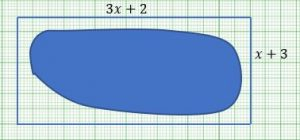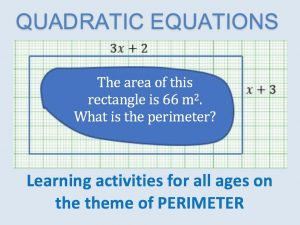1. For security John plans buy the minimum amount of Diamond Razor Mesh fencing material to fence around his dam and discourage entry.  He sent his 15 year old son Mzu to measure the dam.

Mzu tells his Dad that this rectangular shape he has drawn of the dam and fence can help him.

To test his Dad Mzu has given the dimensions in algebraic form, in metres.

1. Find an expression for the area of this rectangle.
2. If the area of the rectangle is 66 m2 , form an equation in x.
3. Transform the equation into the form ax2 +bx + c = 0. State the values of a, b and c.
4. What value of x gives John the information about the length of fence needed? [Hint: solve the quadratic equation].
5. Find the length and width of the rectangular shape surrounding the dam.
6. Find the total perimeter of the fence around the dam.

2. John intends to reserve three (3) metres for putting in a gate. What actual length of the fencing material should John buy?

3. Diamond Razor Mesh fencing material costs R5000.00 for 30 Find the cost of the actual length of fencing material needed?The QUADRATIC EQUATIONS Inclusion and Home Learning Guide offers related learning tasks on the theme of Perimeter suitable for learners of all ability levels and all ages from 5 to 18+.